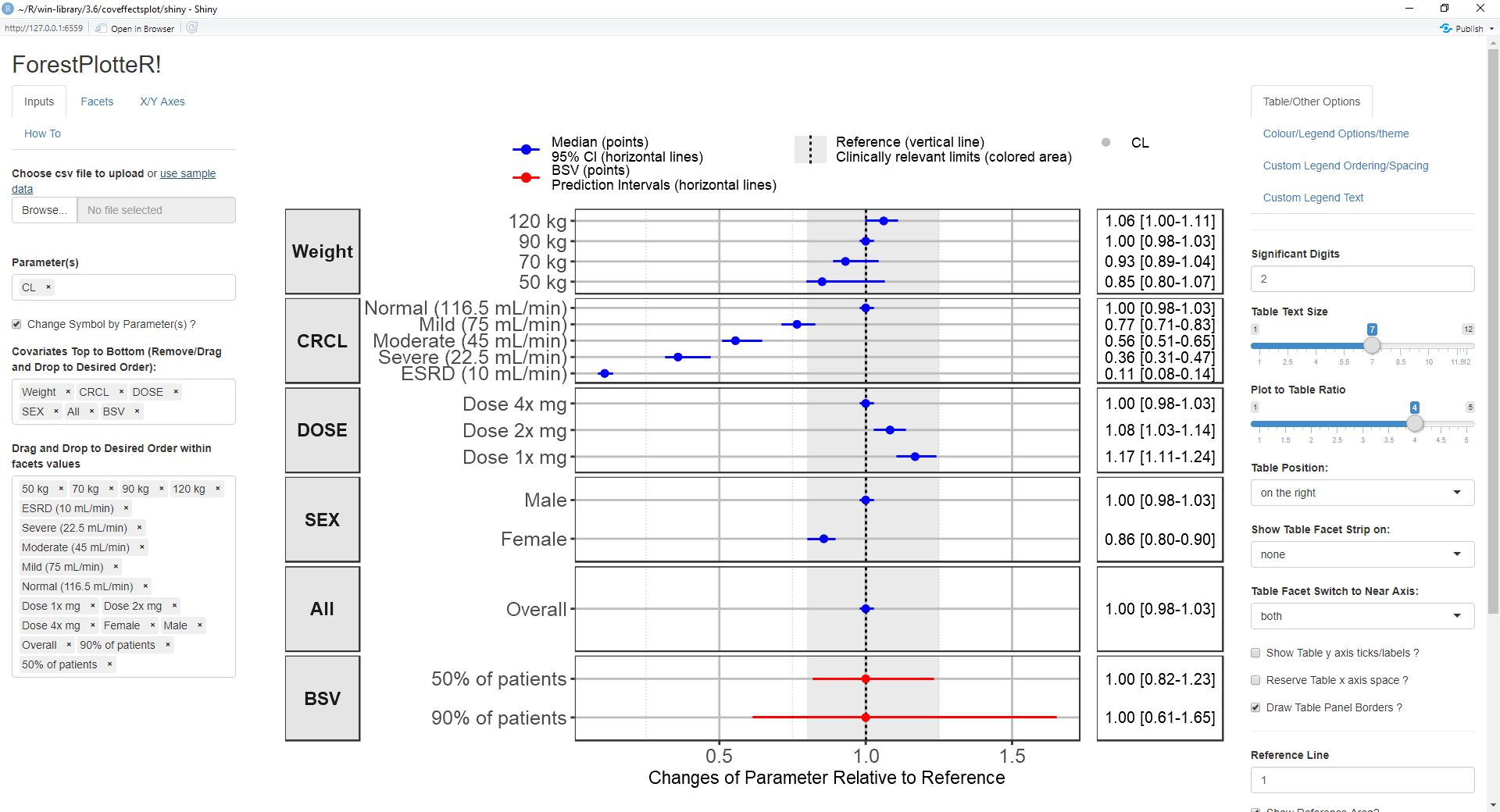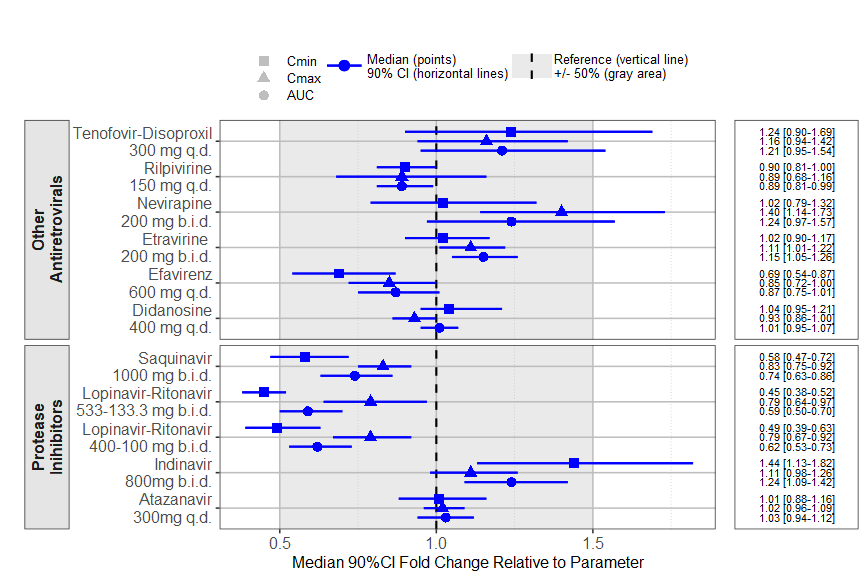Produce Forest Plots to Visualize Covariate Effects

Produce forest plots to visualize covariate effects using either the command line or an interactive 'Shiny' application.

coveffectsplotA function and a Shiny App that Produce Forest Plots to Visualize Covariate Effects as commonly used in pharmacometrics population PK/PD reports.

``````# Install from CRAN:
install.packages("coveffectsplot")
# Or the development version from GitHub:
#install.packages("devtools")
devtools::install_github('smouksassi/coveffectsplot')
``````

Launch the app using this command then press the button to load the example data:

``````coveffectsplot::run_interactiveforestplot()
``````

This will generate this plot:Expected data

Several example data are provided to illustrate the various functionality but the goal is that you bring your own data. It should have at a minimum the following columns with the exact names:

paramname: Parameter on which the effects are shown e.g. CL, Cmax, AUC etc.
covname: Covariate name that the effects belong to e.g. Weight, SEX, Dose etc.
label: Covariate value that the effects of which is shown e.g. 50 kg, 50 kg\90 kg (here the reference value is contained in the label).
mid: Middle value for the effects usually the median from the uncertainty distribution.
lower: Lower value for the effects usually the 2.5% or 5% from the uncertainty distribution.
upper: Upper value for the effects usually the 97.5% or 95% from the uncertainty distribution.

You might also choose to have a covname with value All (or other appropriate value) to illustrate and show the uncertainty on the reference value in a separate facet.

Additionally, you might want to have a covname with value BSV to illustrate and show the the between subject variability (BSV) spread.

The example data show where does 90 and 50% of the patients will be based on the model BSV estimate for the selected paramname(s).

The vignette Introduction to coveffectsplot will walk you through the background and how to compute and build the required data that the shiny app or the function `forest_plot`expects. There is some data management steps that the app does automatically. Choosing to call the function will expect that you will take the responsibility to build the table LABEL and to control the ordering of the variables.

Example using a real drug label data

The prezista drug label data was extracted from the FDA label and calling the `forest_plot` function gives:

``````require(coveffectsplot)
plotdata <- dplyr::mutate(prezista,
LABEL = paste0(format(round(mid,2), nsmall = 2),
" [", format(round(lower,2), nsmall = 2), "-",
format(round(upper,2), nsmall = 2), "]"))
plotdata<- as.data.frame(plotdata)
plotdata<- plotdata[,c("paramname","covname","label","mid","lower","upper","LABEL")]

coveffectsplot::forest_plot(plotdata,
ref_area = c(0.5, 1.5),
x_facet_text_size = 13,
y_facet_text_size = 16,
interval_legend_text = "Median (points)\n90% CI (horizontal lines)",
ref_legend_text = "Reference (vertical line)\n+/- 50% (gray area)",
area_legend_text = "Reference (vertical line)\n+/- 50% (gray area)",
xlabel = "Median 90%CI Fold Change Relative to Parameter",
facet_formula = "covname~.",
facet_switch = "both",
facet_scales = "free",
facet_space = "fixed",
paramname_shape = TRUE,
table_position = "right",
table_text_size = 4,
plot_table_ratio = 4,
vertical_dodge_height = 0.8,
legend_space_x_mult = 0.1,
legend_order = c("ref", "area", "shape"),
show_table_facet_strip = "none",
return_list = FALSE)
``````coveffectsplot 0.0.3

• added an option to return a list of plots to enable further modification to the ggplot(s) if need be
• updated the vignette and examples to demo the new options
• added possibility to choose on which facet to show strips for the table
• fixed a bug that showed up with ggplot dev version by explicitly defining a data.frame to the data argument
• minor ui tweaks to enable named colors in colourpicker

coveffectsplot 0.0.2

• Removed reference to the old name of the package
• updated vignette and docs
• tweaked the order of the shape legends to reverse
• modified default height of vertical dodging and added an option
• added an option to control legend item x spacing

coveffectsplot 0.0.1

• Initial Release of coveffectsplot

Reference manual

install.packages("coveffectsplot")

0.0.3 by Samer Mouksassi, 4 months ago

https://github.com/smouksassi/interactiveforestplot

Report a bug at https://github.com/smouksassi/interactiveforestplot/issues

Browse source code at https://github.com/cran/coveffectsplot

Authors: Samer Mouksassi [aut, cre] , Dean Attali [ctb]

Documentation:   PDF Manual